# Analog & Digital Signals

To send the digital data over an analog media, it needs to be converted into analog signal.There can be two cases according to data formatting.

Bandpass:The filters are used to filter and pass frequencies of interest. A bandpass is a band of frequencies which can pass the filter.

Low-pass: Low-pass is a filter that passes low frequencies signals.

When digital data is converted into a bandpass analog signal, it is called digital-to-analog conversion. When low-pass analog signal is converted into bandpass analog signal, it is called analog-to-analog conversion.

## Digital-to-Analog Conversion

When data from one computer is sent to another via some analog carrier, it is first converted into analog signals. Analog signals are modified to reflect digital data.

An analog signal is characterized by its amplitude, frequency, and phase. There are three kinds of digital-to-analog conversions:

• Amplitude Shift Keying

In this conversion technique, the amplitude of analog carrier signal is modified to reflect binary data.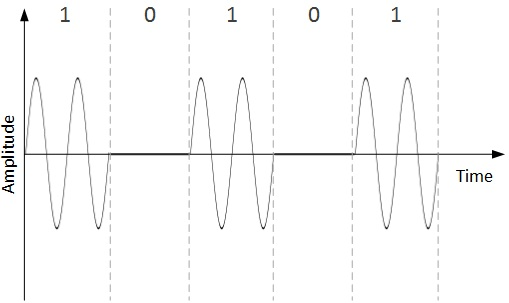When binary data represents digit 1, the amplitude is held; otherwise it is set to 0. Both frequency and phase remain same as in the original carrier signal.

• Frequency Shift Keying

In this conversion technique, the frequency of the analog carrier signal is modified to reflect binary data.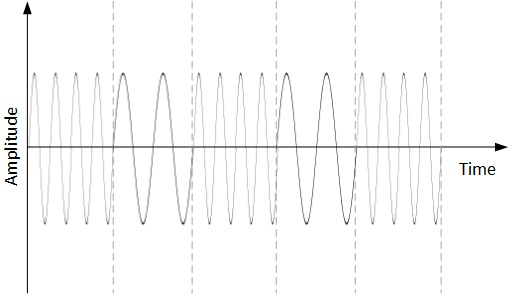This technique uses two frequencies, f1 and f2. One of them, for example f1, is chosen to represent binary digit 1 and the other one is used to represent binary digit 0. Both amplitude and phase of the carrier wave are kept intact.

• Phase Shift Keying

In this conversion scheme, the phase of the original carrier signal is altered to reflect the binary data.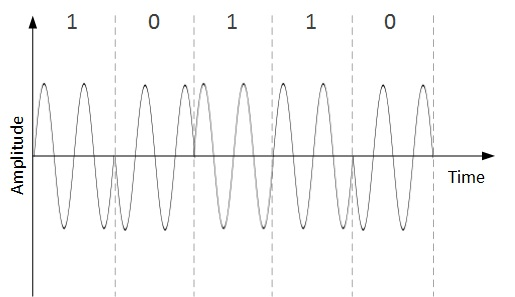When a new binary symbol is encountered, the phase of the signal is altered. Amplitude and frequency of the original carrier signal is kept intact.

• Quadrature Phase Shift Keying

QPSK alters the phase to reflect two binary digits at once. This is done in two different phases. The main stream of binary data is divided equally into two sub-streams. The serial data is converted in to parallel in both sub-streams and then each stream is converted to digital signal using NRZ technique. Later, both the digital signals are merged together.

## Analog-to-Analog Conversion

Analog signals are modified to represent analog data. This conversion is also known as Analog Modulation. Analog modulation is required when bandpass is used. Analog to analog conversion can be done in three ways: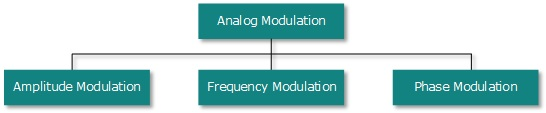• Amplitude Modulation

In this modulation, the amplitude of the carrier signal is modified to reflect the analog data.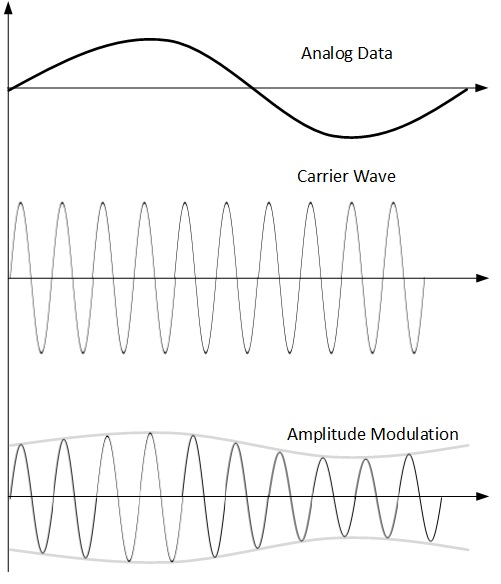Amplitude modulation is implemented by means of a multiplier. The amplitude of modulating signal (analog data) is multiplied by the amplitude of carrier frequency, which then reflects analog data.

The frequency and phase of carrier signal remain unchanged.

• Frequency Modulation

In this modulation technique, the frequency of the carrier signal is modified to reflect the change in the voltage levels of the modulating signal (analog data).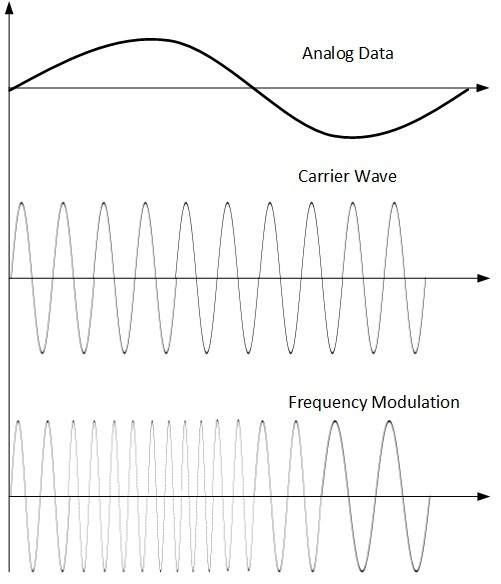The amplitude and phase of the carrier signal are not altered.

• Phase Modulation

In the modulation technique, the phase of carrier signal is modulated in order to reflect the change in voltage (amplitude) of analog data signal.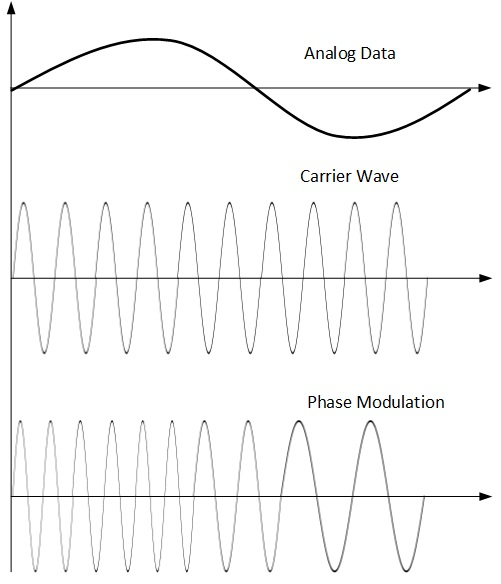Phase modulation is practically similar to Frequency Modulation, but in Phase modulation frequency of the carrier signal is not increased. Frequency of carrier is signal is changed (made dense and sparse) to reflect voltage change in the amplitude of modulating signal.

Data or information can be stored in two ways, analog and digital. For a computer to use the data, it must be in discrete digital form.Similar to data, signals can also be in analog and digital form. To transmit data digitally, it needs to be first converted to digital form.

## Digital-to-Digital Conversion

This section explains how to convert digital data into digital signals. It can be done in two ways, line coding and block coding. For all communications, line coding is necessary whereas block coding is optional.

## Line Coding

The process for converting digital data into digital signal is said to be Line Coding. Digital data is found in binary format.It is represented (stored) internally as series of 1s and 0s.Digital signal is denoted by discreet signal, which represents digital data.There are three types of line coding schemes available:### Uni-polar Encoding

Unipolar encoding schemes use single voltage level to represent data. In this case, to represent binary 1, high voltage is transmitted and to represent 0, no voltage is transmitted. It is also called Unipolar-Non-return-to-zero, because there is no rest condition i.e. it either represents 1 or 0.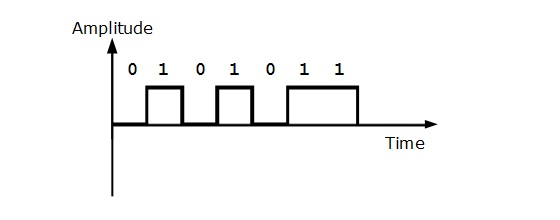### Polar Encoding

Polar encoding scheme uses multiple voltage levels to represent binary values. Polar encodings is available in four types:

• Polar Non-Return to Zero (Polar NRZ)

It uses two different voltage levels to represent binary values. Generally, positive voltage represents 1 and negative value represents 0. It is also NRZ because there is no rest condition.

NRZ scheme has two variants: NRZ-L and NRZ-I.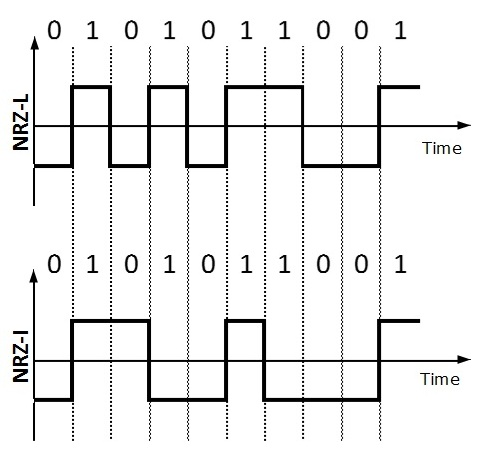NRZ-L changes voltage level at when a different bit is encountered whereas NRZ-I changes voltage when a 1 is encountered.

Problem with NRZ is that the receiver cannot conclude when a bit ended and when the next bit is started, in case when sender and receiver’s clock are not synchronized.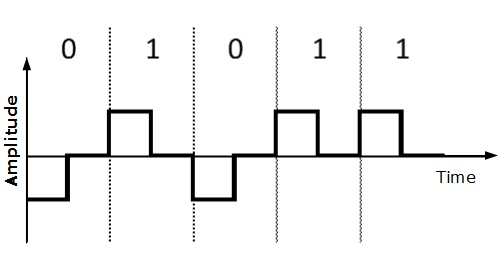RZ uses three voltage levels, positive voltage to represent 1, negative voltage to represent 0 and zero voltage for none. Signals change during bits not between bits.

• ### Manchester

This encoding scheme is a combination of RZ and NRZ-L. Bit time is divided into two halves. It transits in the middle of the bit and changes phase when a different bit is encountered.

• ### Differential Manchester

This encoding scheme is a combination of RZ and NRZ-I. It also transit at the middle of the bit but changes phase only when 1 is encountered.

### Bipolar Encoding

Bipolar encoding uses three voltage levels, positive, negative and zero. Zero voltage represents binary 0 and bit 1 is represented by altering positive and negative voltages.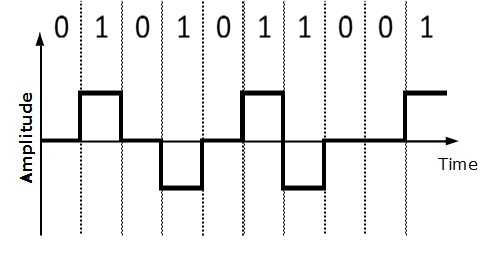## Block Coding

To ensure accuracy of the received data frame redundant bits are used. For example, in even-parity, one parity bit is added to make the count of 1s in the frame even. This way the original number of bits is increased. It is called Block Coding.

Block coding is represented by slash notation, mB/nB.Means, m-bit block is substituted with n-bit block where n > m. Block coding involves three steps:

• Division,
• Substitution
• Combination.

After block coding is done, it is line coded for transmission.

## Analog-to-Digital Conversion

Microphones create analog voice and camera creates analog videos, which are treated is analog data. To transmit this analog data over digital signals, we need analog to digital conversion.

Analog data is a continuous stream of data in the wave form whereas digital data is discrete. To convert analog wave into digital data, we use Pulse Code Modulation (PCM).

PCM is one of the most commonly used method to convert analog data into digital form. It involves three steps:

• Sampling
• Quantization
• Encoding.

### Sampling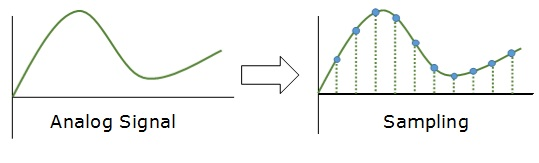The analog signal is sampled every T interval. Most important factor in sampling is the rate at which analog signal is sampled. According to Nyquist Theorem, the sampling rate must be at least two times of the highest frequency of the signal.

### Quantization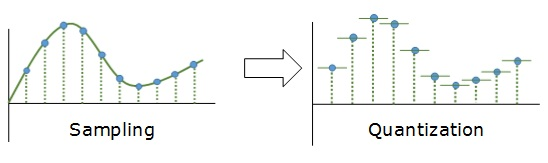Sampling yields discrete form of continuous analog signal. Every discrete pattern shows the amplitude of the analog signal at that instance. The quantization is done between the maximum amplitude value and the minimum amplitude value. Quantization is approximation of the instantaneous analog value.

### Encoding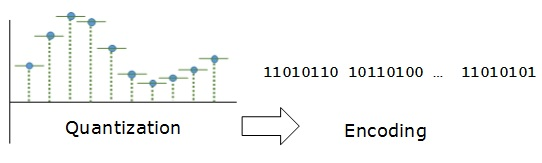In encoding, each approximated value is then converted into binary format.

## Transmission Modes

The transmission mode decides how data is transmitted between two computers.The binary data in the form of 1s and 0s can be sent in two different modes: Parallel and Serial.

### Parallel Transmission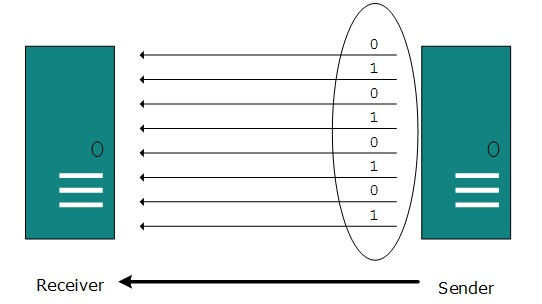The binary bits are organized in-to groups of fixed length. Both sender and receiver are connected in parallel with the equal number of data lines. Both computers distinguish between high order and low order data lines. The sender sends all the bits at once on all lines.Because the data lines are equal to the number of bits in a group or data frame, a complete group of bits (data frame) is sent in one go. Advantage of Parallel transmission is high speed and disadvantage is the cost of wires, as it is equal to the number of bits sent in parallel.

### Serial Transmission

In serial transmission, bits are sent one after another in a queue manner. Serial transmission requires only one communication channel.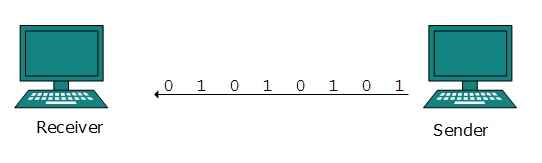Serial transmission can be either asynchronous or synchronous.

### Asynchronous Serial Transmission

It is named so because there’is no importance of timing. Data-bits have specific pattern and they help receiver recognize the start and end data bits.For example, a 0 is prefixed on every data byte and one or more 1s are added at the end.

Two continuous data-frames (bytes) may have a gap between them.

### Synchronous Serial Transmission

Timing in synchronous transmission has importance as there is no mechanism followed to recognize start and end data bits.There is no pattern or prefix/suffix method. Data bits are sent in burst mode without maintaining gap between bytes (8-bits). Single burst of data bits may contain a number of bytes. Therefore, timing becomes very important.

It is up to the receiver to recognize and separate bits into bytes.The advantage of synchronous transmission is high speed, and it has no overhead of extra header and footer bits as in asynchronous transmission.

Hi I am Alfred.